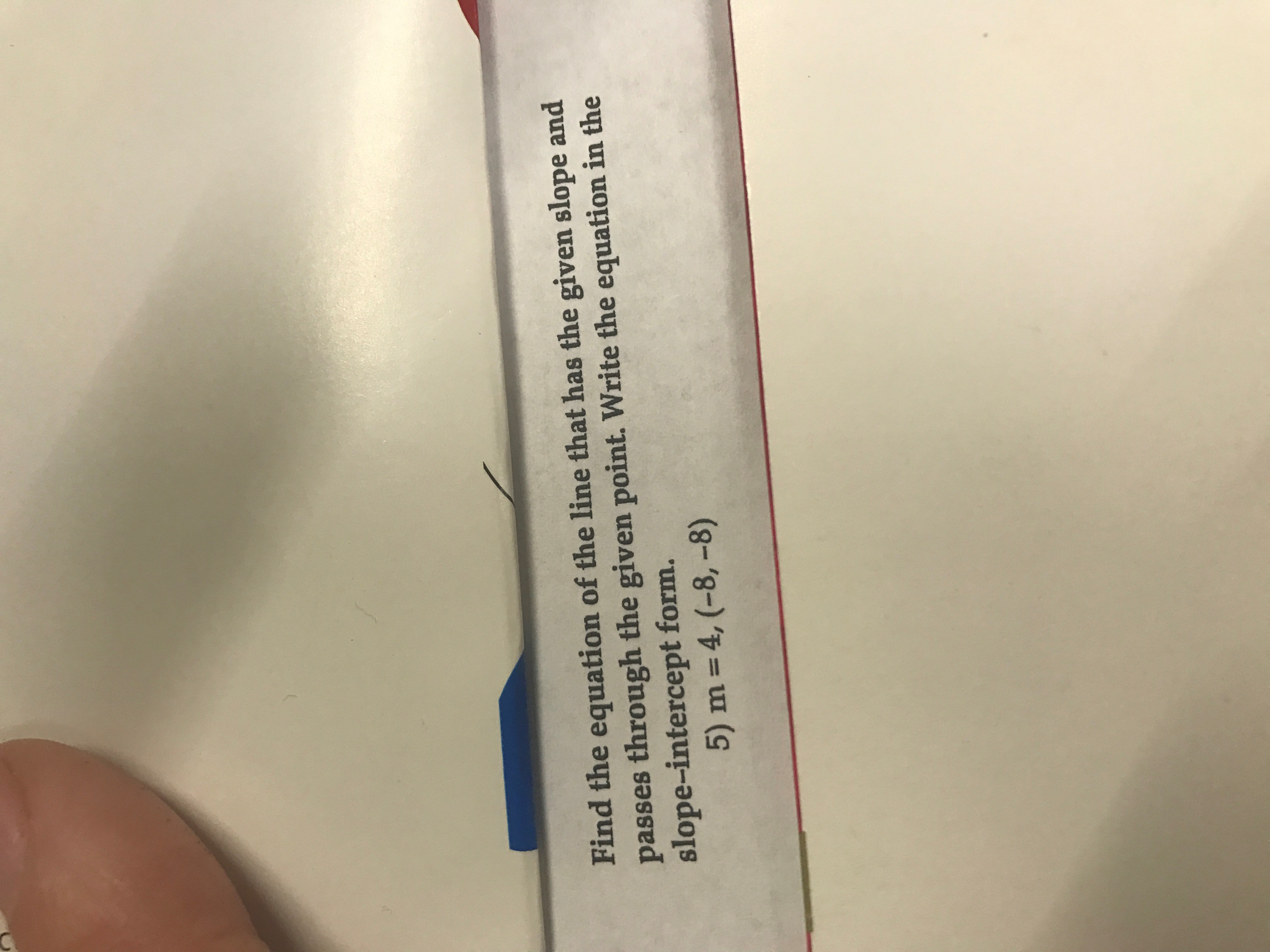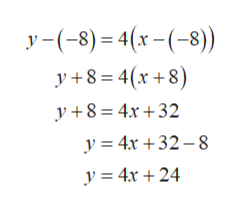# Find the equation of the line that has the given slope andpasses through the given point. Write the equation in theslope-intercept form.5) m4, (-8,-8)

Question
3 viewshelp_outlineImage TranscriptioncloseFind the equation of the line that has the given slope and passes through the given point. Write the equation in the slope-intercept form. 5) m 4, (-8,-8) fullscreen
check_circle

Step 1

The equation of line with slope as m and passing through the point (a,b).

Step 2

Now here m=4 and (a...help_outlineImage Transcriptionclosey-(8)4(x(8) y+8 4(8) y 8 4x+32 y = 4x +32-8 y = 4x 24 fullscreen

### Want to see the full answer?

See Solution

#### Want to see this answer and more?

Solutions are written by subject experts who are available 24/7. Questions are typically answered within 1 hour.*

See Solution
*Response times may vary by subject and question.
Tagged in

### Other